# RD Sharma Solutions For Class 12 Maths Exercise 19.29 Chapter 19 Indefinite Integrals

RD Sharma Solutions for Class 12 Maths Exercise 19.29 Chapter 19 Indefinite Integrals is provided here. RD Sharma Solutions are one of the best resource materials currently available for students who aspire to score well in Maths. These study materials prove that they are essential for the students to succeed in the examination. BYJU’S brings you the RD Sharma Solutions for Class 12 Maths in a convenient downloadable PDF format, which can be quick and easy to access.

These RD Sharma Solutions are structured by BYJU’S experts, which help students to remember the crucial points perfectly. This exercise of Chapter 19 Indefinite Integrals is mainly focused on the integrals of the form

$$\begin{array}{l}\int (px+q) \sqrt{ax^{2}+bx+c}\ dx\end{array}$$
with algorithm.

## Download the PDF of RD Sharma Solutions For Class 12 Chapter 19 – Indefinite Integrals Exercise 19.29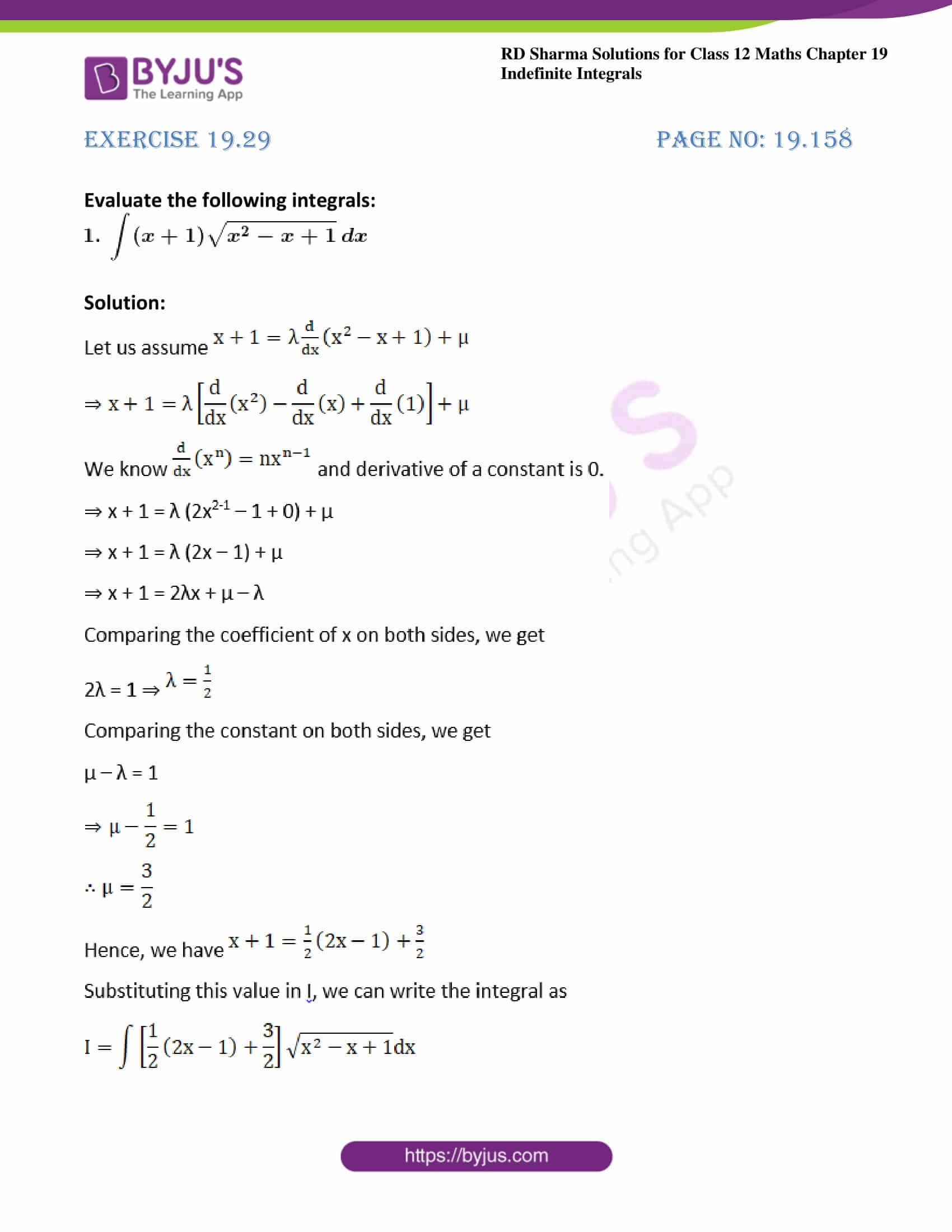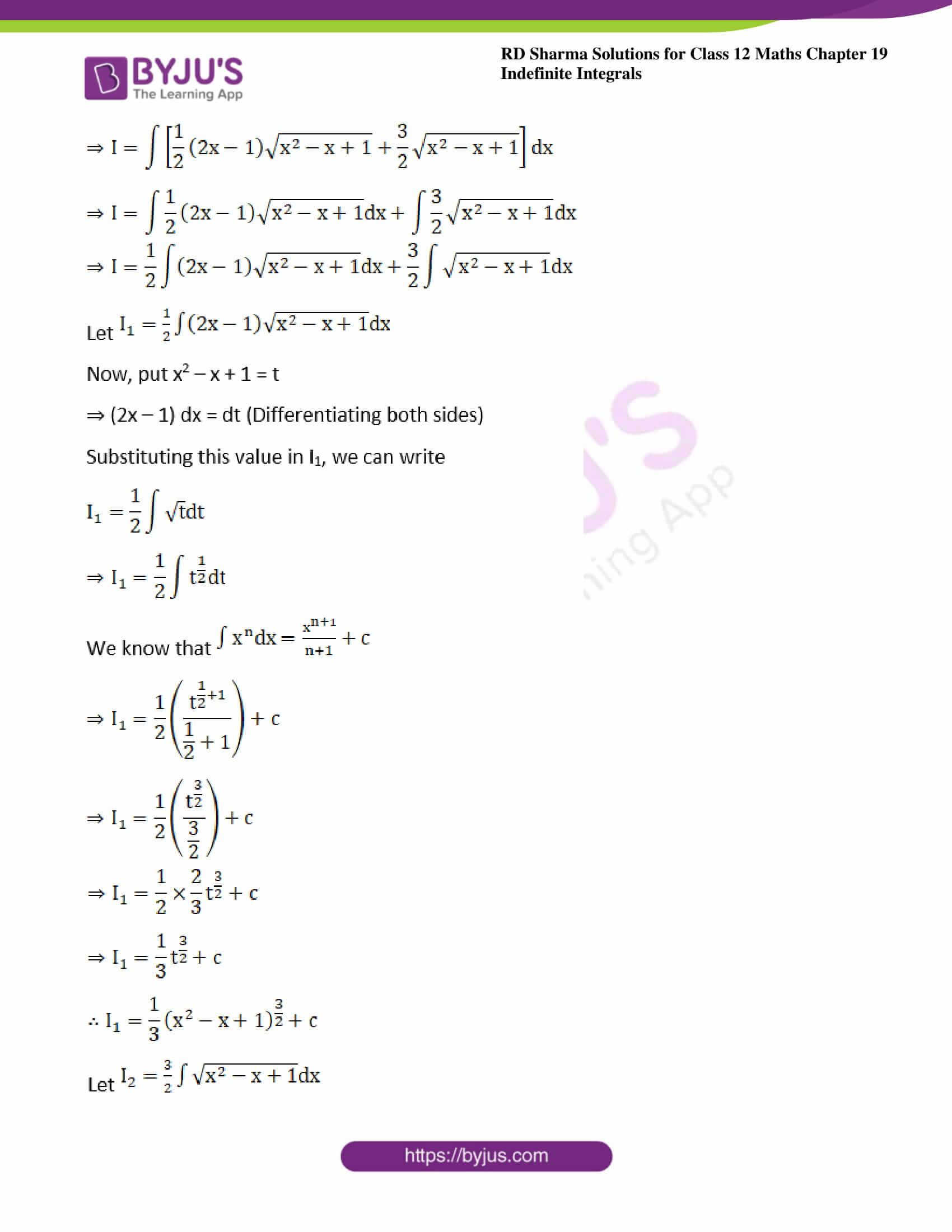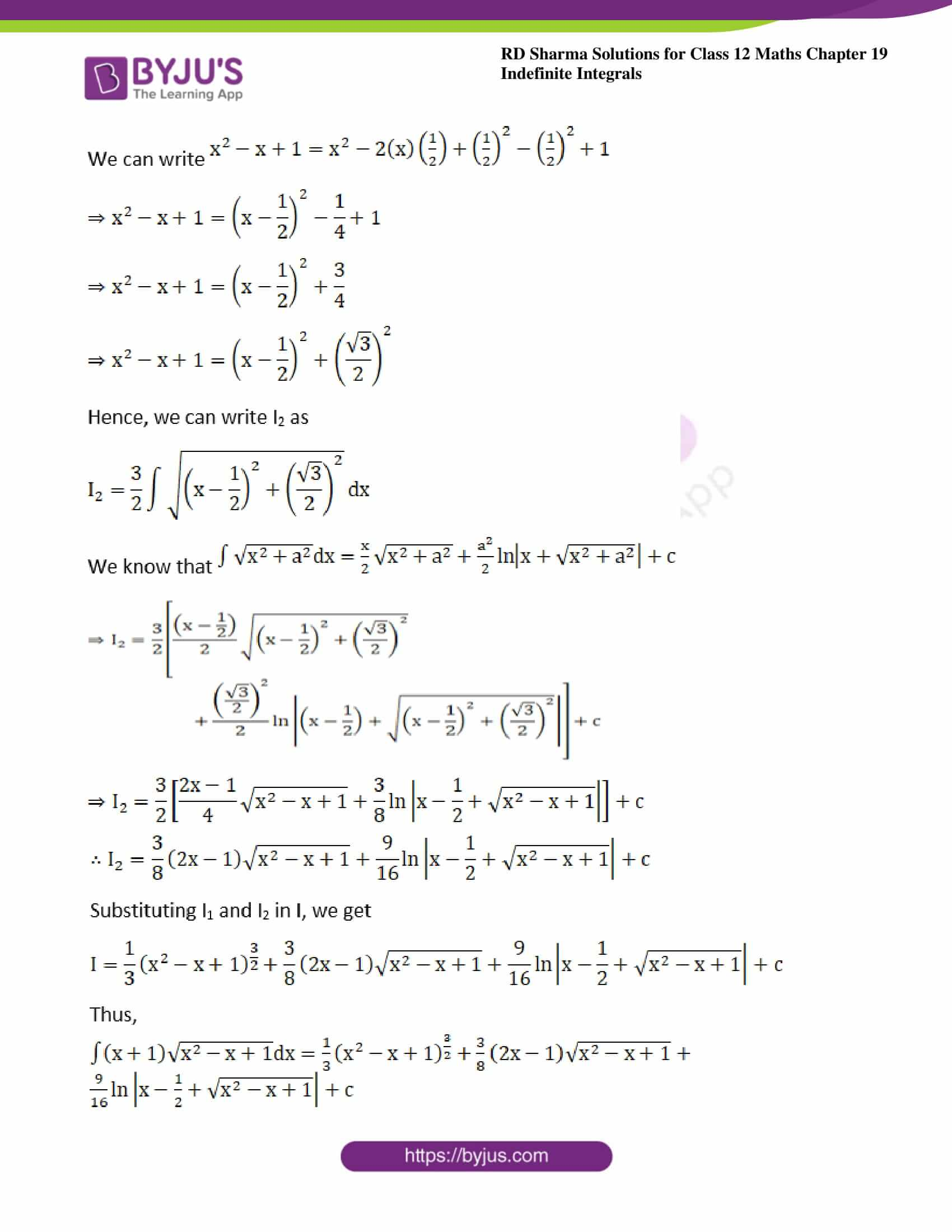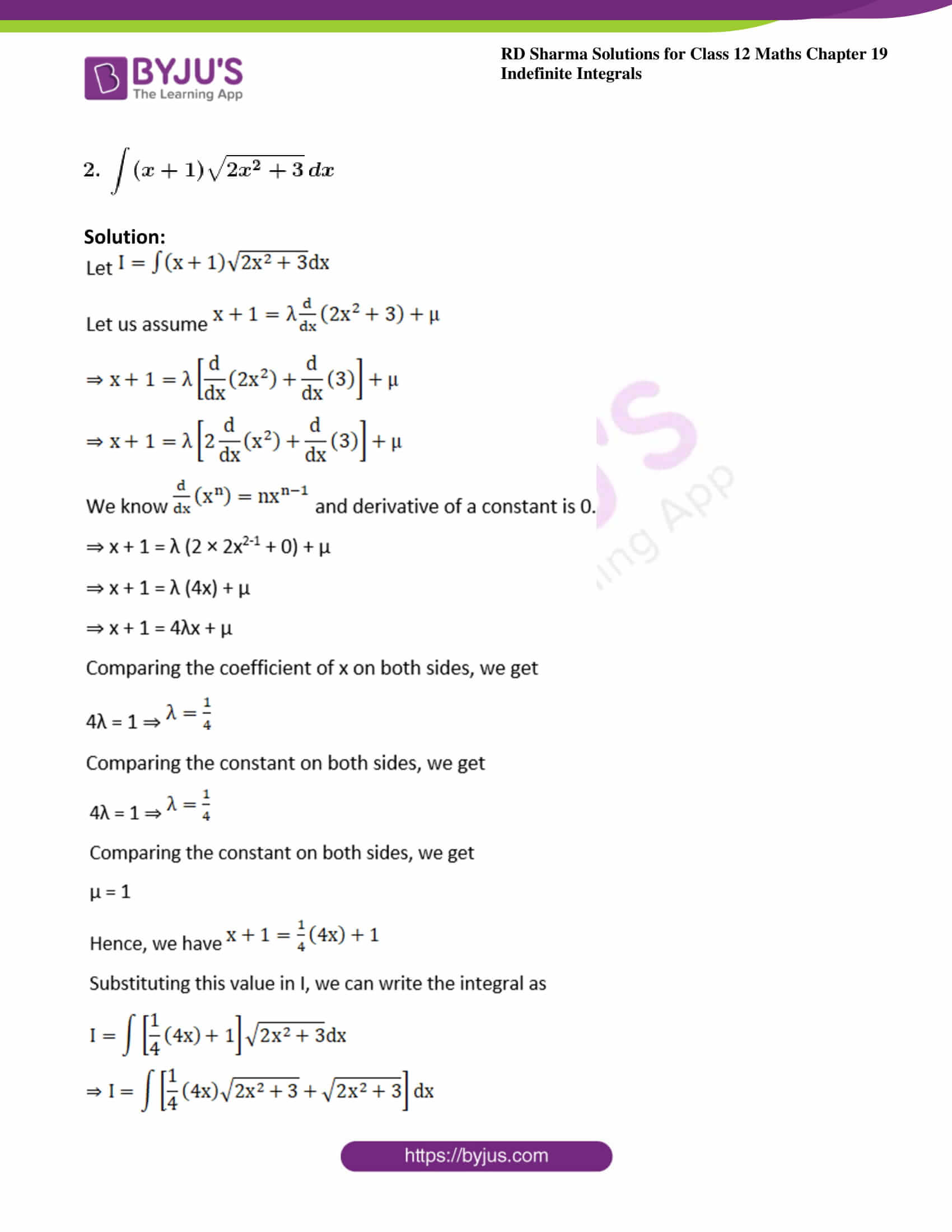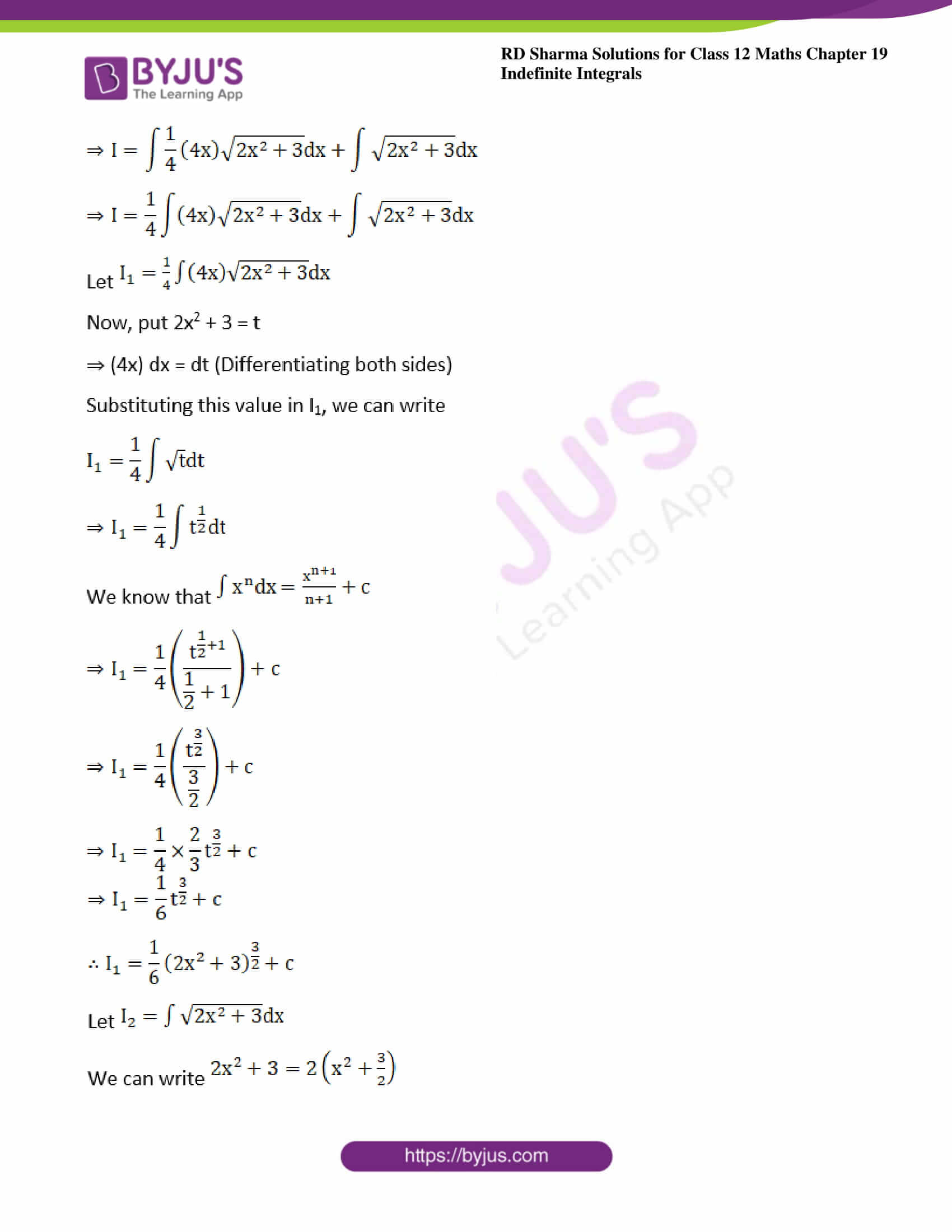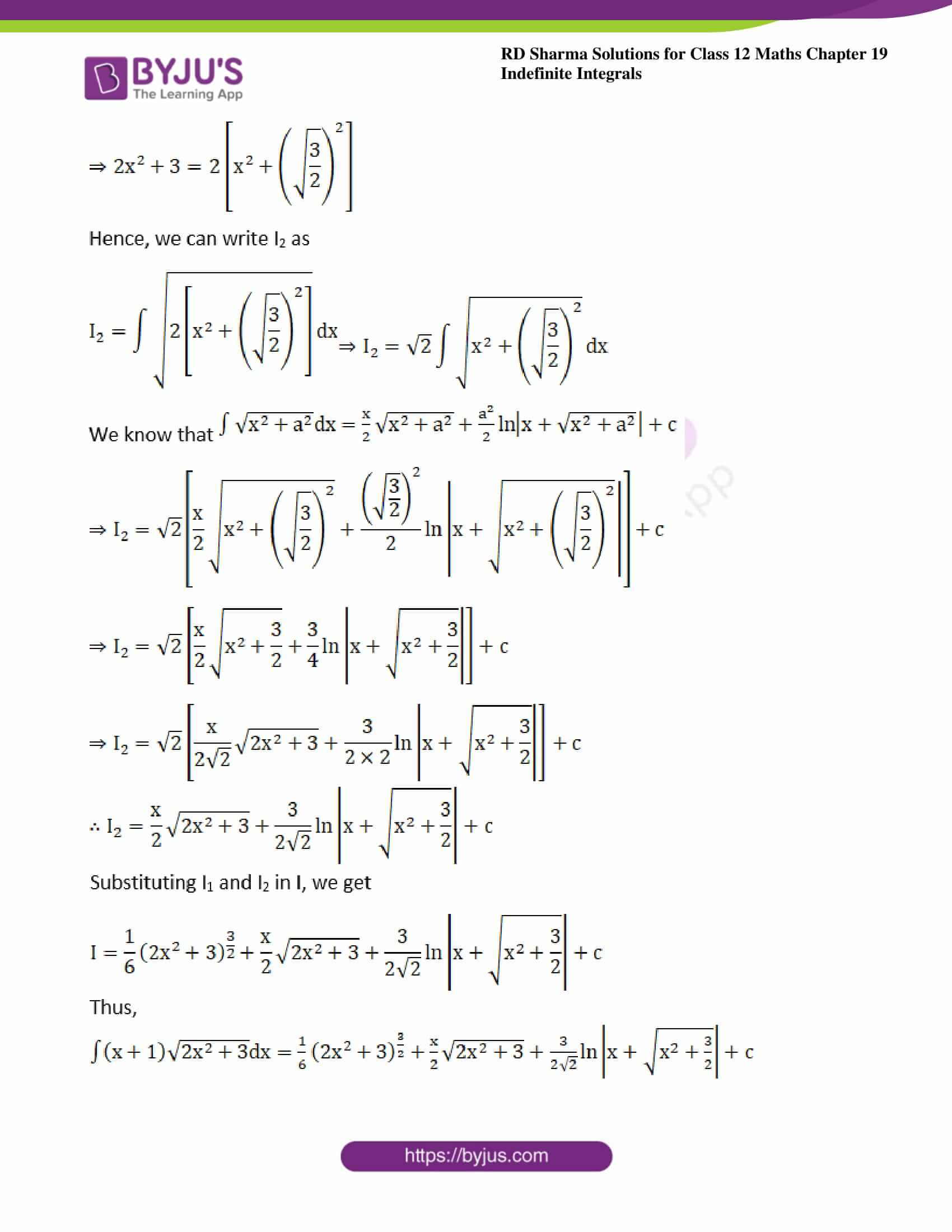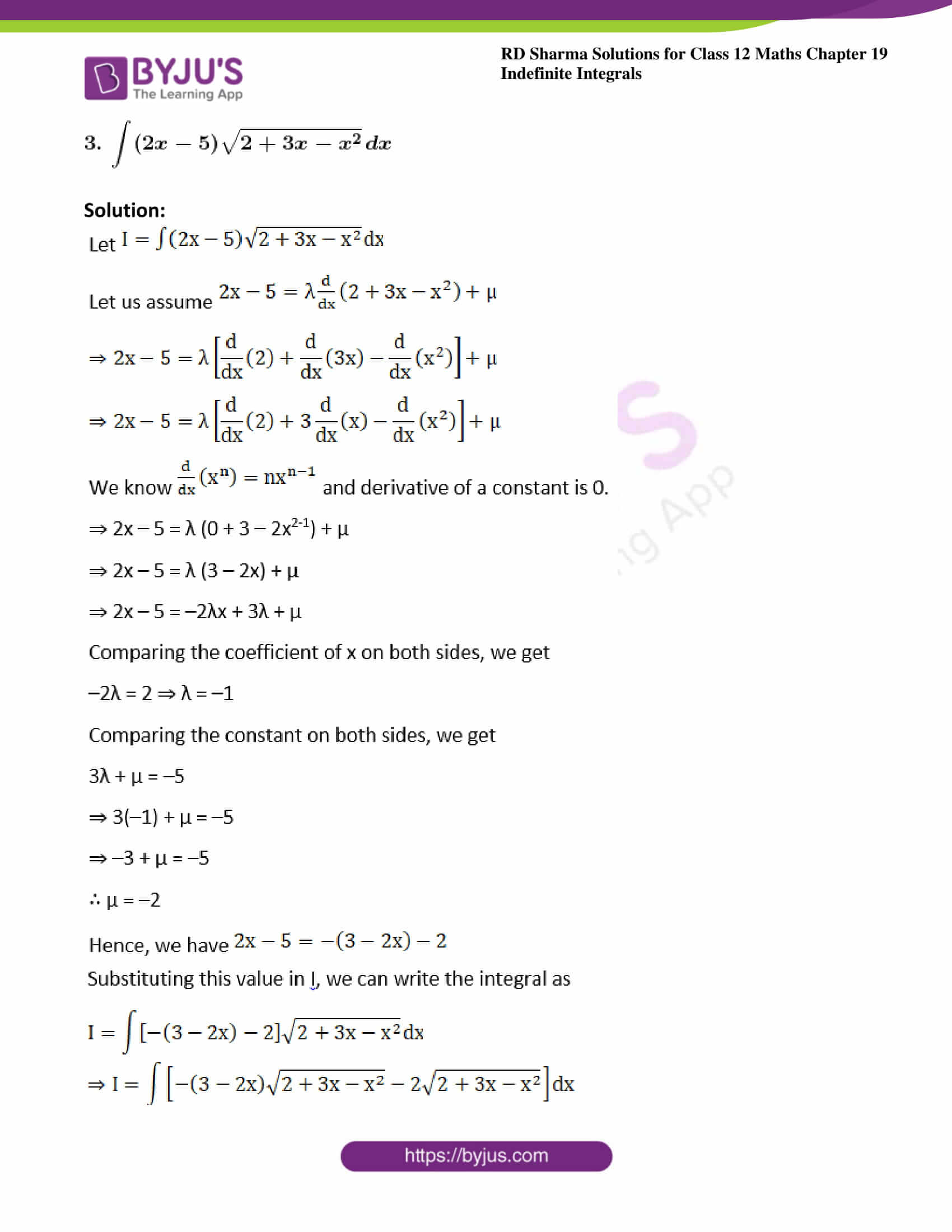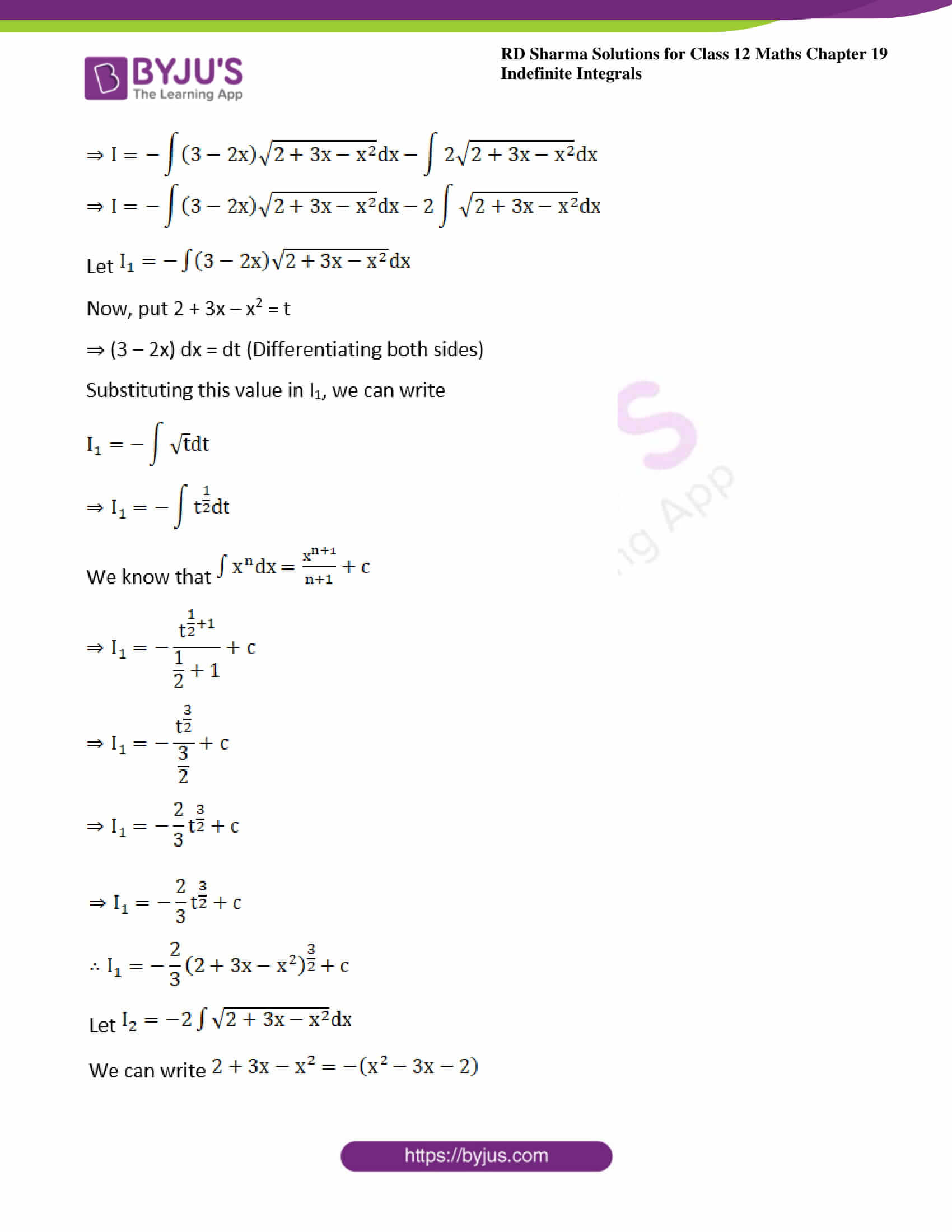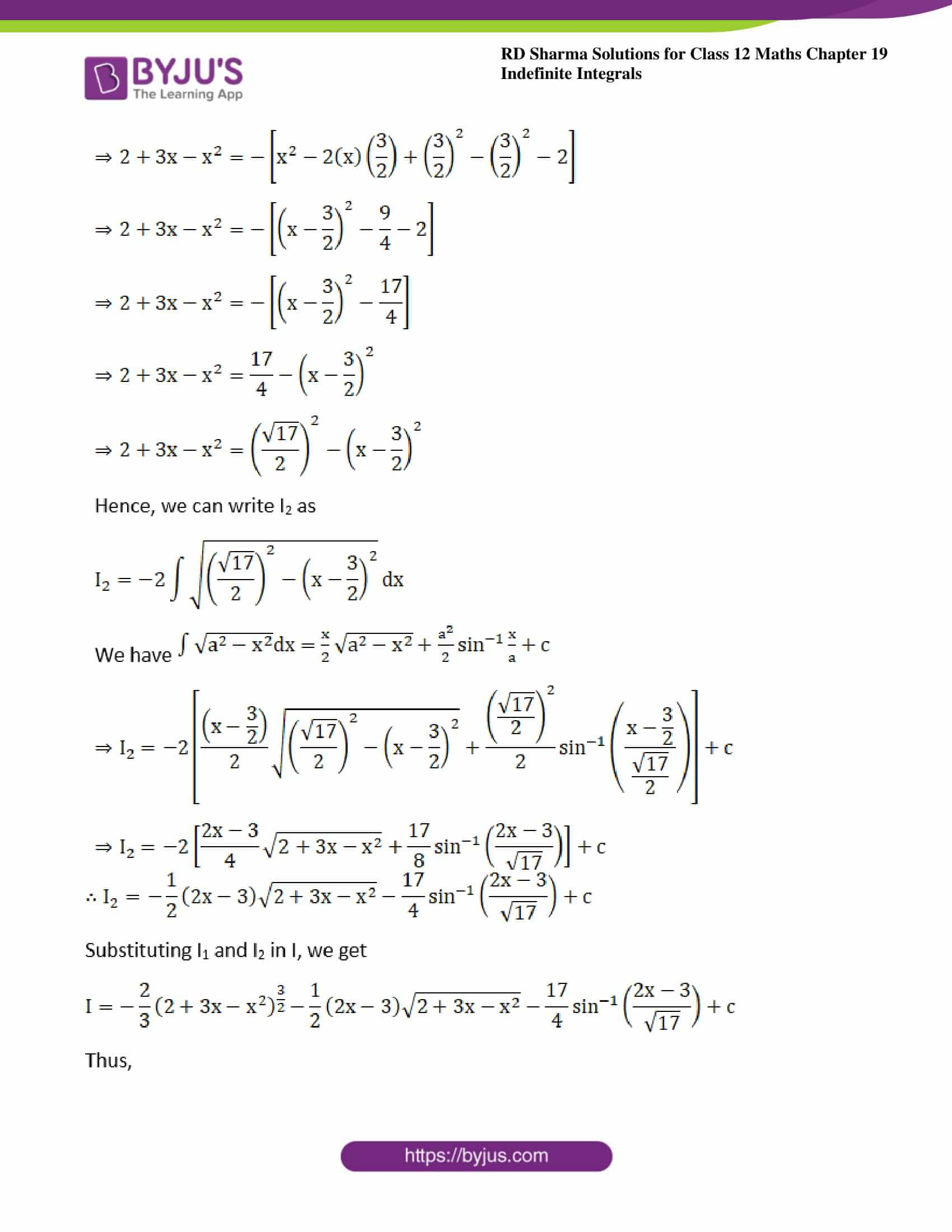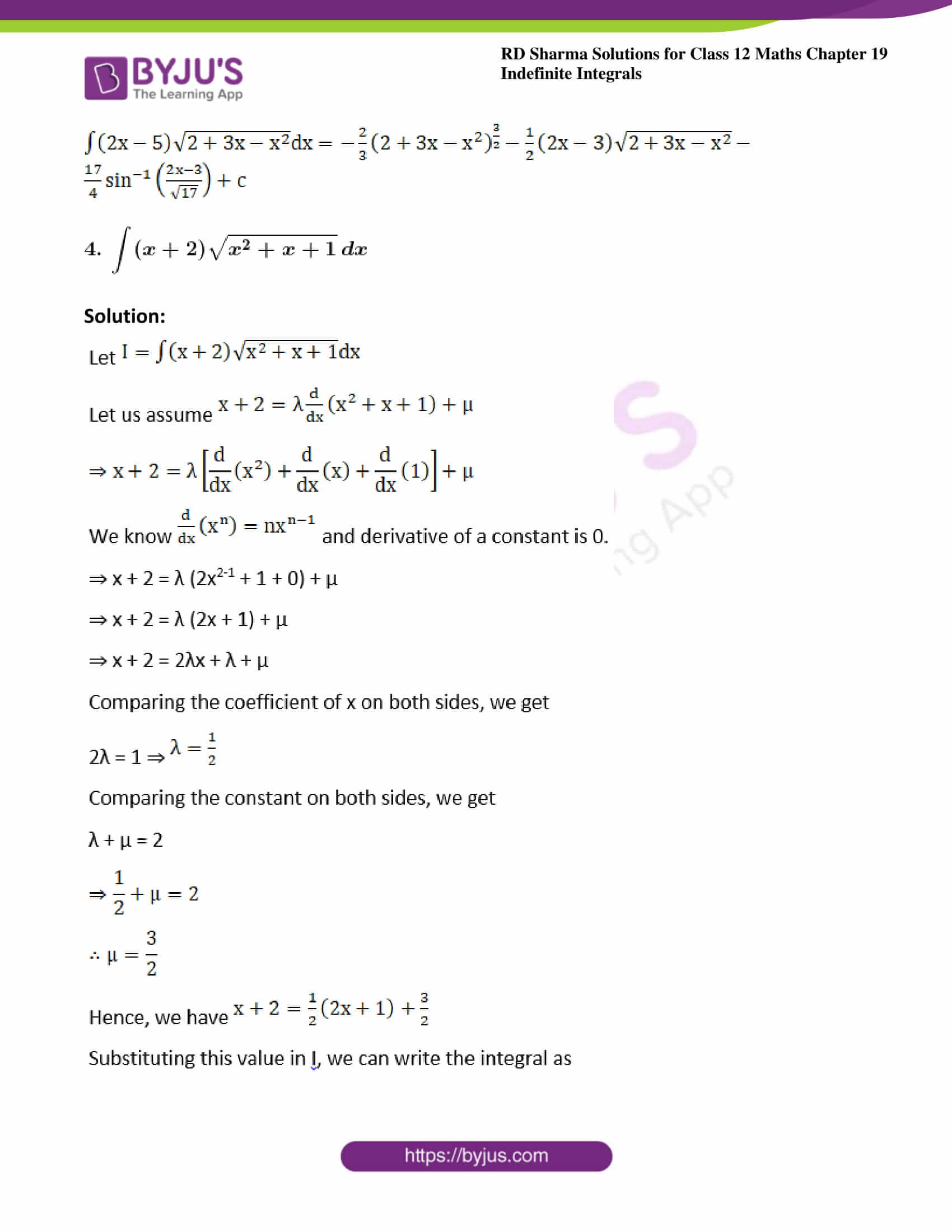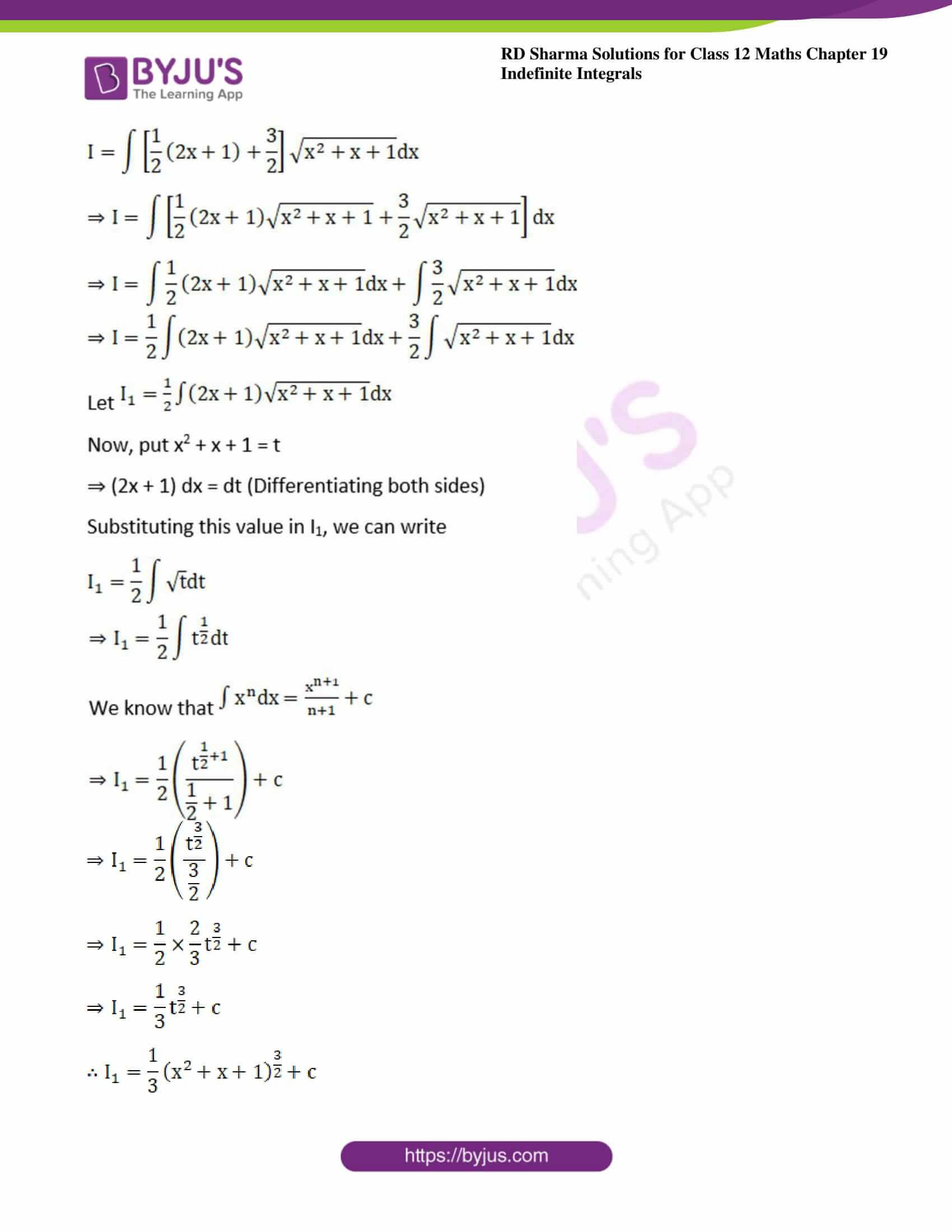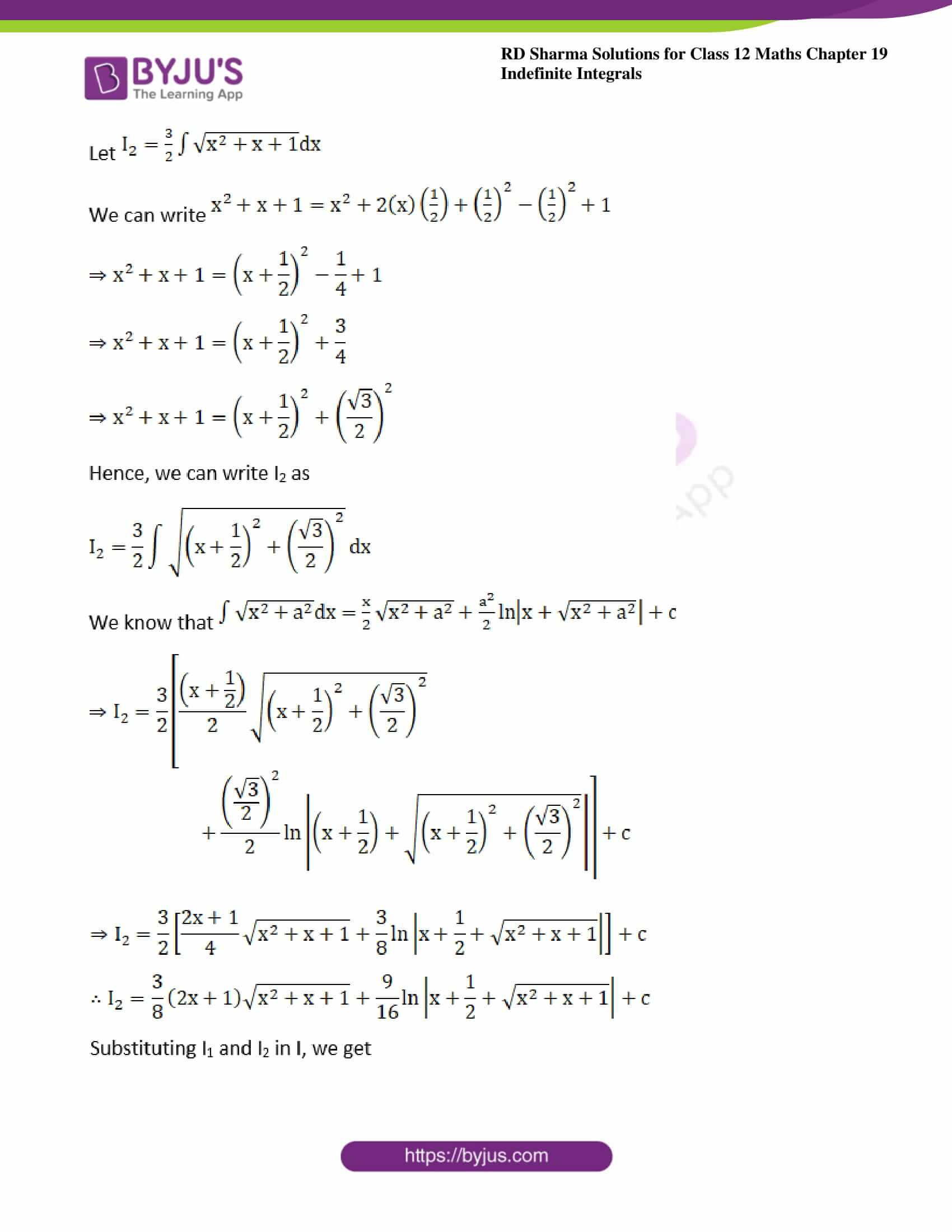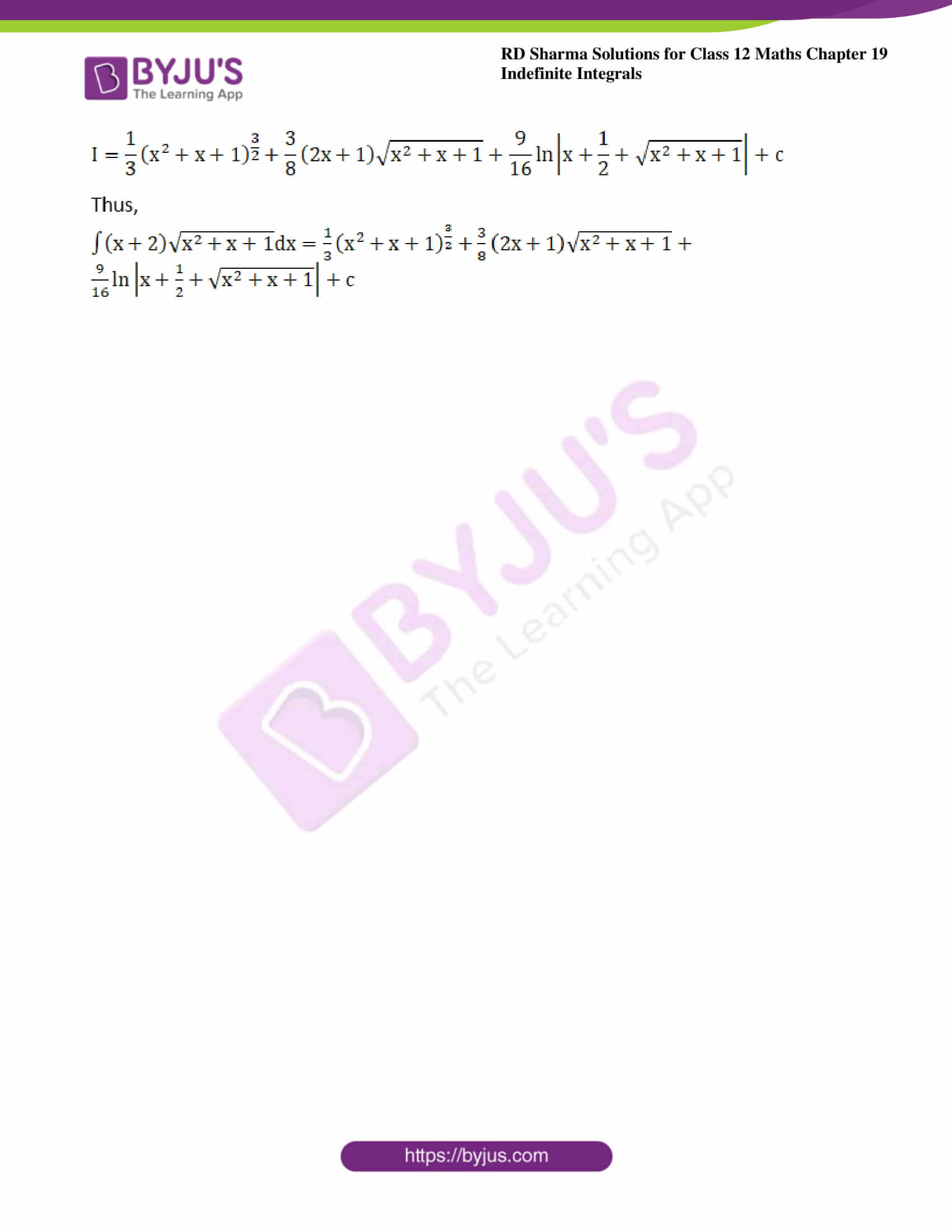### Exercise 19.29 Page No: 19.158

Evaluate the following integrals: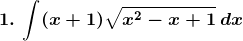Solution: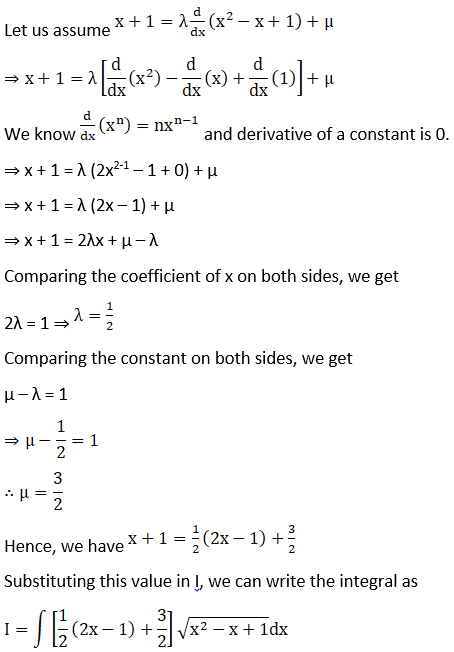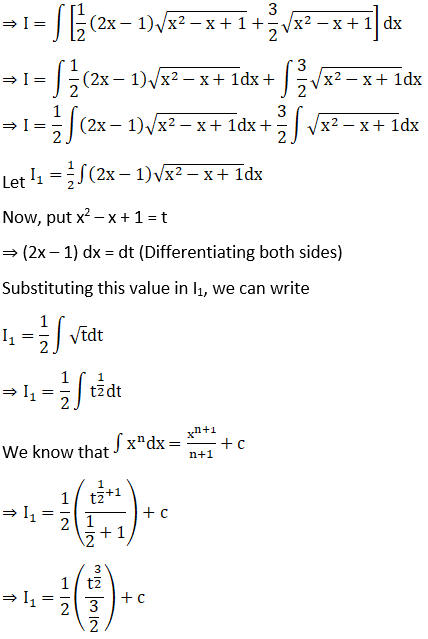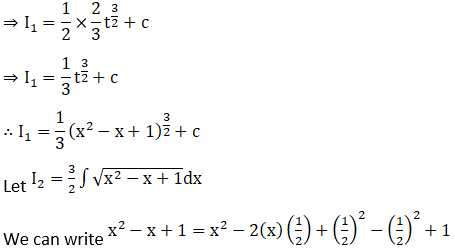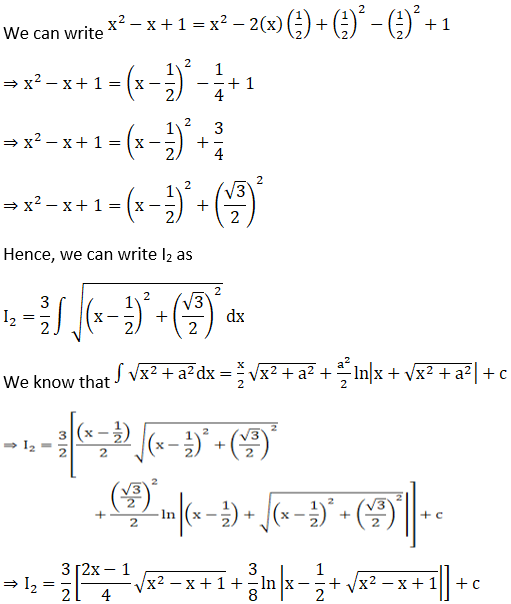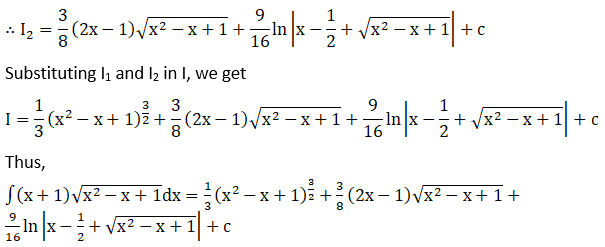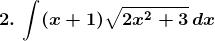Solution: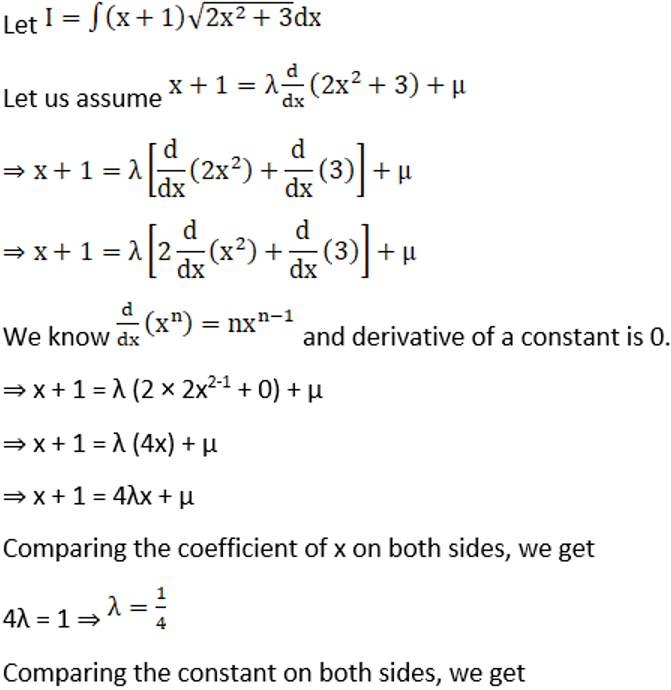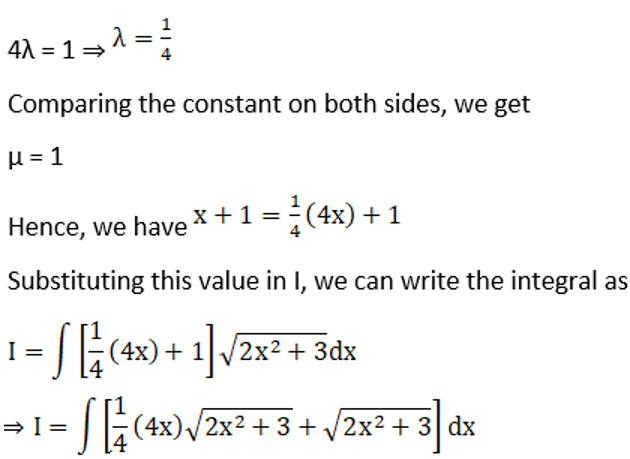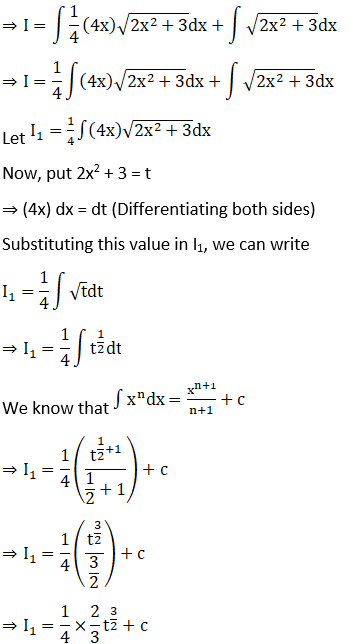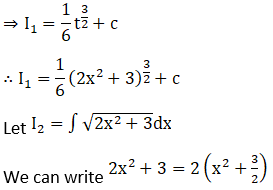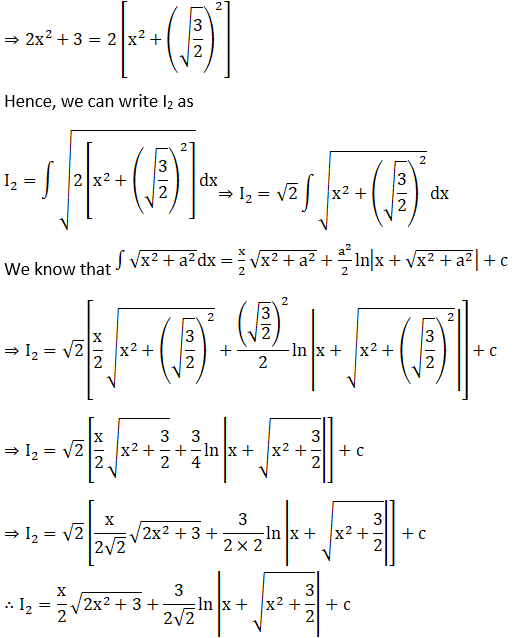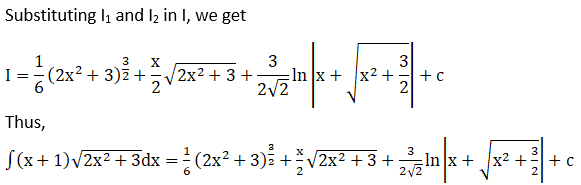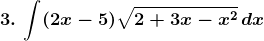Solution: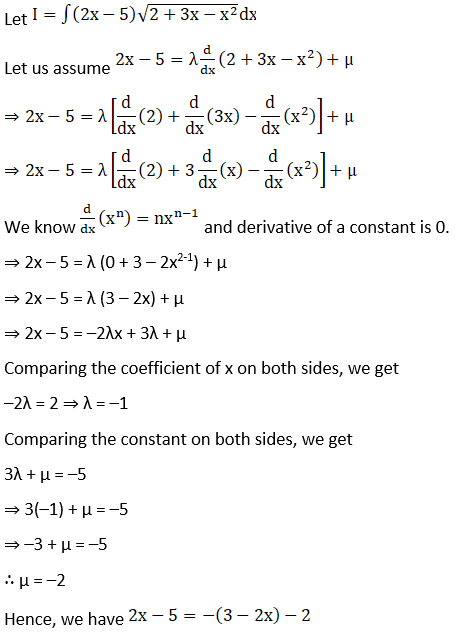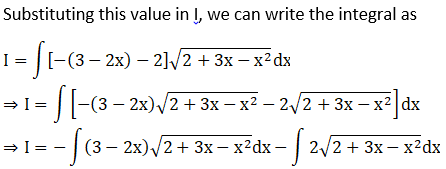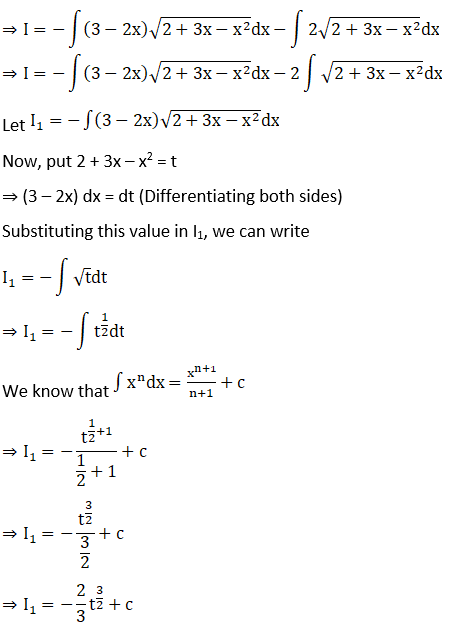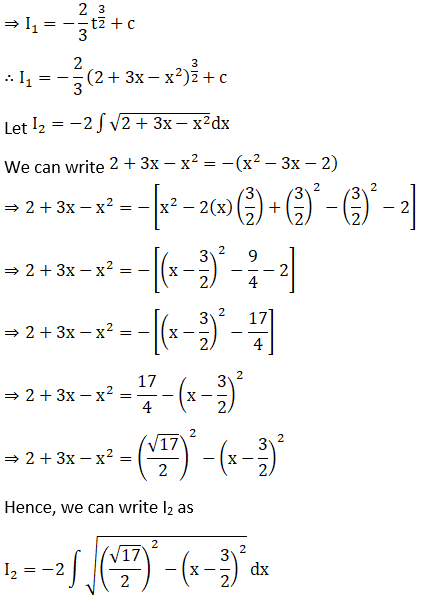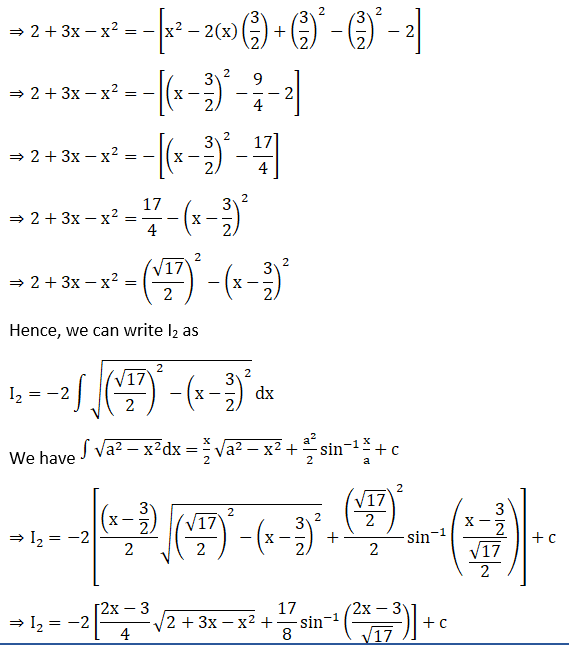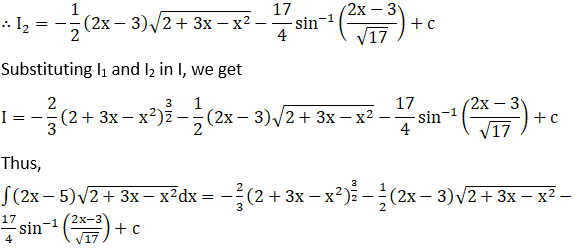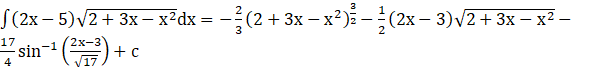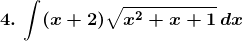Solution: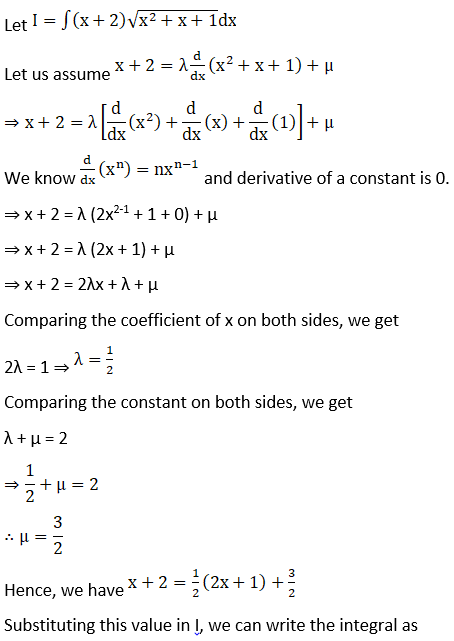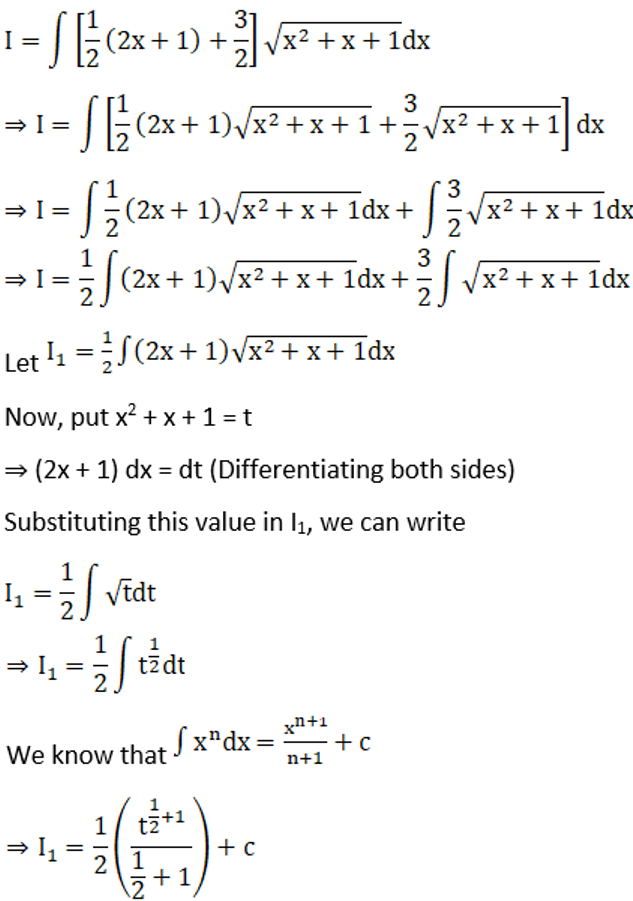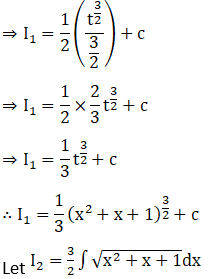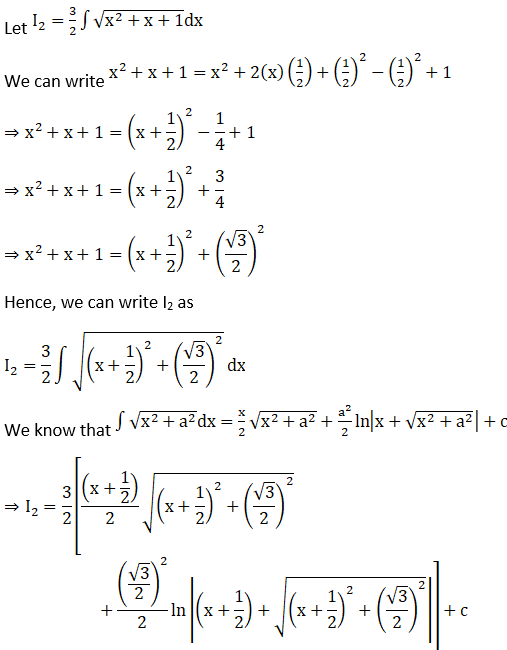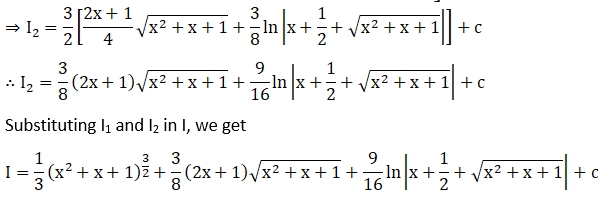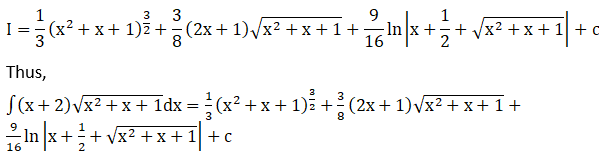### Access other exercises of RD Sharma Solutions For Class 12 Chapter 19 – Indefinite Integrals

Exercise 19.1 Solutions

Exercise 19.2 Solutions

Exercise 19.3 Solutions

Exercise 19.4 Solutions

Exercise 19.5 Solutions

Exercise 19.6 Solutions

Exercise 19.7 Solutions

Exercise 19.8 Solutions

Exercise 19.9 Solutions

Exercise 19.10 Solutions

Exercise 19.11 Solutions

Exercise 19.12 Solutions

Exercise 19.13 Solutions

Exercise 19.14 Solutions

Exercise 19.15 Solutions

Exercise 19.16 Solutions

Exercise 19.17 Solutions

Exercise 19.18 Solutions

Exercise 19.19 Solutions

Exercise 19.20 Solutions

Exercise 19.21 Solutions

Exercise 19.22 Solutions

Exercise 19.23 Solutions

Exercise 19.24 Solutions

Exercise 19.25 Solutions

Exercise 19.26 Solutions

Exercise 19.27 Solutions

Exercise 19.28 Solutions

Exercise 19.30 Solutions

Exercise 19.31 Solutions

Exercise 19.32 Solutions